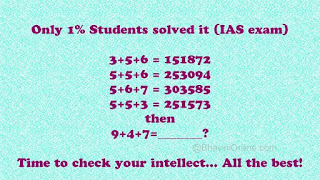SOLUTION OF THIS PUZZLE

The solution to the first 4 digits is pretty straight forward, and I am sure most of you must have easily got it.

It works out to be a+b+c = (a*b)(a*c)??

The last 2 digits are really tough to crack…

Here’s how you do it. Reverse of {(a×b)+(a×c)-c}

So the number would (a×b),(a×c),{(a×b)+(a×c)-c}reverse.

So for 1st one: 3+5+6=151872;

axb = 3×5 = 15; axc = 3×6 = 18;

{(a×b)+(a×c)-c} = ((3×5)+(3×6)-6} = 27

— Reverse of 27 is 72

So 9+4+7=_______?

axb = 9×4 = 36;

axc = 9×7 = 63;

{(a×b)+(a×c)-c} = ((9×4)+(9×7)-7} = (36+63-7)=92

— Reverse of 92 is 29.

Answer :::: Hence 9+4+7 = 366329

આ પણ વાંચો  Enjoy The jigsaw puzzle, its realy amazing and so funny